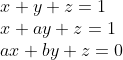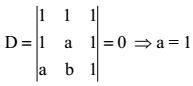# Basis maths-linear algebra-Q15

+1 vote

If S is set of distinct values of b for which the system of linear equations,has no solution, then S is :

(A). A Singleton

(B). An Empty Set

(C). An Infinite Set

(D). A Finite Set Containing Two or More Elementsasked May 15
reshown May 16

Here,For a = 1, the equations become

x + y + z = 1

x + y + z = 1

x + by + z = 0

These equations give no solution for b = 1

Therefore, S is singleton set.answered May 16 by (112,390 points)